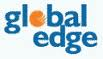# Placement Papers - GlobaledgeGlobal Edge - Placement Paper Pattern
Posted by :
Achchhelal
(295)
Global Edge Placement Paper

1) what is big-endian.
c) memory mgmt technique
d) none of the above
Ans:a

2)what is Little-endian.
c) memory mgmt technique
d) none of the above
Ans:b

3)8086 has
a)16 bit data bus ,16 bit address bus
b)16 bit data bus,32 bit address bus
c)8 bit data bus,16 bit address bus
d)8 bit data bus,8 bit address bus
Ans:a

4) what is the scheduling algorithm used in general operating systems.
a) FCFS algorithm
b) Highest Priority First algorithm
c) Round-Robin algorithm
d) None of the above
Ans:c

5)Router is present at
a)Physical layer
c)Network Layer
d)None of above
Ans:c

a) Mutual Exclusion
b) no premption
c) hold and wait
d) circular wait
e) all of the above
Ans:d

7)PCI stands for
a)Programmable computer Interface
b)Peripheral Computer Interface
c)programmable Control Interface
d)Peripheral Component Interface
Ans:d

8)Toggle state in J-K flip-flop is
a)0 1
b)1 0
c)1 1
d)0 0
Ans :c

9)Interrupt is serviced
a)Immediatly when it occurs
b)After the completion of current instruction.
c)Ignored
d)None of the above.
Ans:b

C Questions :

1.what is the o/p ?

void main()
{
char *mess[]={"Have","a","nice","day","Bye");
printf("%d %d",sizeof(mess),sizeof(mess));
}
a. 16 4
b. 5 4
c. 20 4
d. Error

2.what is the o/p of the following programe?
void main()
{
int i,count=0;
char *p1="abcdefghij";
char *p2="alcmenfoip";

for(i=0;i<=strlen(p1);i++) {
if(*p1++ == *p2++)
count+=5;
else
count-=3;
}
printf("count=%d
",count);
}

a. 15
b. 6
c. 12
d. compiler error
Answer: b ( note : strlen(p1) == i when i=6)

3.what does main return on successful execution?
a. 1
b. 0
c. -1
d.Nonzero

4.
main(int argc,char *argv[])
{
printf((argc > 1 ? "%c" : "%c",*++argv);
}
If the i/p string is "GESL Bangalore".
a. G
b. E
c. B
d. GESL

5. How do u declare a pointer to an array of pointers to int?
a. int *a;
b. int **a;
c. int *(*a);
d. u con not declare

Questions on OS:

1.UDP is a
a. Reliable protocol
b. Unreliable protocol
c. Connectionless protocol
d. Both b & c

2.Real Time OS uses
a. RISC processor
b. CISC processor
c. SISC processor
d. Any of the above

3.Race condition could be overcome using
a. A global variable
b. A local variable
c. Mutex
d. All of the above

4.Repeaters are in
b. Physical layer
c. Network layer
d. Transport layer